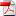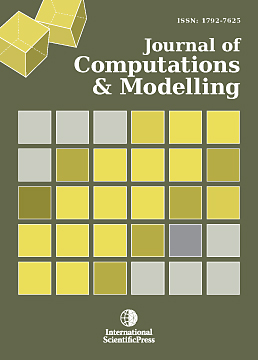# Journal of Computations & Modelling

#### Variational assimilation method for solving an optimal control problem to identify initial height of dune in an aquatic environment

•[ Download ]
• Times downloaded: 501
• Abstract

This paper concerns the estimation of the optimal initial dune height which can favor the evolution of the dune in the depth of an aquatic environment in two space dimension. The problem is formulated as an optimal control problem, governed by nonlinear equations describing the dune height formation. The control is done in a discrete framework, where the model solution gap to the data is minimized using a quasi-Newton method. The functional gradient is computed by the backward time integration of the corresponding discrete adjoint model. Numerical tests are provided in order to discuss the eﬃciency and the eﬀectiveness of our approach.

Mathematics Subject Classiﬁcation: 49J20, 47J35, 76D05, 74G65, 49M15.

Keywords: Optimal control, nonlinear evolution equations, incompressible ﬂows, minimization, quasi-Newton method, discrete adjoint method.ISSN: 1792-8850(Online)
1792-7625 (Print)